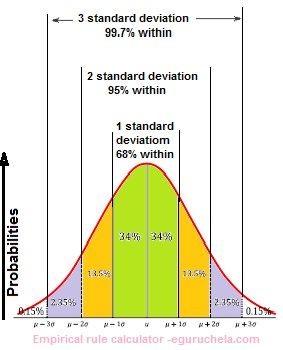# Empirical Rule Calculation

Find the Empirical rule for the given set of data using this calculator.

Total Numbers
Mean (Average)
Standard Deviation
Empirical Rule at 68% falls between
Empirical Rule at 95% falls between
Empirical Rule at 97.7% falls between

Empirical rule is the statistical rule for a normal distribution determined with the mean and the standard deviation.

According to it, 68 % of data falls within first SD, 95 % within first two SD and 99.7 % within first three SD's.

Hence it is also known as 68-95-99.7 or three sigma rule.

The empirical rule works because it allows to estimate probabilities very quickly when someone dealing with a normal distribution.

We often create ranges using standard deviation, so knowing what percentage of cases fall within 1, 2 and 3 standard deviations can be useful.The empirical rule referred as three-sigma rule or 68-95-99.7 rule, it is a statistical rule which states that for a normal distribution, almost all observed data will fall within three standard deviations (denoted by σ) of the mean or average (denoted by µ).

The empirical value is :
a. derived from or relating to experiment and observation rather than theory,
b. based on practical experience rather than scientific proof and

c. (Philosophy) a (of knowledge) derived from experience rather than by logic from first principles.

Empirical means “based on observation” : The empirical rule come from observations. The Normal/Gaussian distribution is the most common type of data distribution. All of the measurements are computed as distances from the mean and are reported in standard deviations.